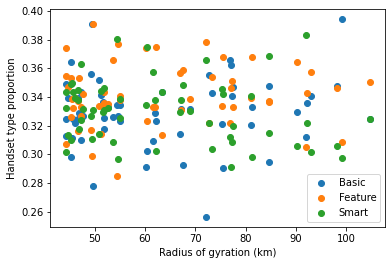# Joined spatial aggregate¶

## Comparing mobility with handset types¶

This worked example demonstrates using joined spatial aggregate queries in FlowKit. A joined spatial aggregate query calculates a metric for each subscriber, and joins the metric values to subscribers' locations before aggregating the metric by region.

Suppose we want to investigate whether there is a link between subscribers' mobility and their handset types. We can calculate two joined spatial aggregates:

• Average radius of gyration per region. Radius of gyration is a measure of the spread of a subscriber's event locations - a subscriber with a large radius of gyration will have events spread over a larger area (for example, somebody who commutes over a long distance or regularly travels to regions away from their home).
• Distribution of handset types (basic/feature/smartphone) per region. Once we have the results of these queries, we can investigate whether there is any correlation between the metrics.

The Jupyter notebook for this worked example can be downloaded here, or can be run using the quick start setup.

### Load FlowClient and connect to FlowAPI¶

We start by importing FlowClient. We also import pandas, geopandas and matplotlib, which we will use for performing further analysis and visualisation of the outputs from FlowKit.

``````import os

import pandas as pd
import geopandas as gpd
import matplotlib.pyplot as plt

import flowclient as fc

%matplotlib inline
``````

We must next generate a FlowAPI access token using FlowAuth. If you are running this notebook using the quick start setup, generating a token requires the following steps:

1. Visit the FlowAuth login page at http://localhost:9091.
2. Log in with username `TEST_USER` and password `DUMMY_PASSWORD`.
3. Under "My Servers", select `TEST_SERVER`.
4. Click the `+` button to create a new token.
5. Give the new token a name, and click `SAVE`.
6. Copy the token string using the `COPY` button.
7. Paste the token in this notebook as `TOKEN`.

The steps are the same in a production setup, but the FlowAuth URL, login details and server name will differ.

Once we have a token, we can start a connection to the FlowAPI system. If you are connecting to FlowAPI over https (recommended) and the system administrator has provided you with an SSL certificate file, you should provide the path to this file as the `ssl_certificate` argument to `flowclient.connect()` (in this example, you can set the path in the environment variable `SSL_CERTIFICATE_FILE`). If you are connecting over http, this argument is not required.

``````conn = fc.connect(
url=os.getenv("FLOWAPI_URL", "http://localhost:9090"),
token=TOKEN,
ssl_certificate=os.getenv("SSL_CERTIFICATE_FILE"),
)
``````

### Specify FlowKit queries¶

Next, we create query specifications for the FlowKit queries we will run.

#### Locations¶

Joined spatial aggregate queries join a per-subscriber metric to the subscribers' locations, so we need a query that assigns a single location to each subscriber. Here we use a modal location query to estimate subscribers' home locations over the period of interest (first seven days of 2016), at administrative level 3.

``````date_range = ("2016-01-01", "2016-01-08")
modal_loc = fc.modal_location_from_dates_spec(
start_date=date_range,
end_date=date_range,
method="last",
)
``````

We create a joined spatial aggregate query specification to calculate the average radius of gyration per region, using locations from the modal location query above.

``````rog_query = fc.radius_of_gyration_spec(
start_date=date_range,
end_date=date_range,
)

jsa_rog_query = fc.aggregates.joined_spatial_aggregate_spec(
locations=modal_loc,
metric=rog_query,
method="avg",
)
``````

#### Handset type¶

Finally, we specify a second joined spatial aggregate query, this time using a `handset` metric with the `"hnd_type"` (handset type) characteristic. The `handset` query will return a categorical variable (handset type is one of "Basic", "Feature" or "Smart"), so we must choose the `"distr"` method for the joined spatial aggregate, to get the distribution of handset types per region.

``````handset_query = fc.handset_spec(
start_date=date_range,
end_date=date_range,
characteristic="hnd_type",
method="most-common",
)

jsa_handset_query = fc.aggregates.joined_spatial_aggregate_spec(
locations=modal_loc,
metric=handset_query,
method="distr",
)
``````

Now that we have a FlowClient connection and we have specified our queries, we can run the queries and get the results using FlowClient's `get_result` function. First, we get the results of the radius of gyration query.

``````rog_results = fc.get_result(connection=conn, query_spec=jsa_rog_query)
``````

pcod value
0 NPL.1.3.3_1 49.1169
1 NPL.4.1.1_1 75.0083
2 NPL.1.1.3_1 48.1
3 NPL.3.1.4_1 107.041
4 NPL.1.2.1_1 54.3766

The resulting dataframe has two columns: `pcod` (the P-code of each region) and `value` (the average radius of gyration, in km). Later, we will want to join with other results for the same regions, so let's set the `pcod` column as the index. We also rename the `value` column, to give it the more descriptive name `radius_of_gyration`.

``````rog_results = (
.set_index("pcod")
)
``````

pcod

NPL.1.3.3_1 49.1169
NPL.4.1.1_1 75.0083
NPL.1.1.3_1 48.1
NPL.3.1.4_1 107.041
NPL.1.2.1_1 54.3766

We can get a summary of the data using the dataframe's `describe` method, and quickly see the distribution of values using `plot.hist`.

``````rog_results.describe()
``````
count 60
mean 64.0353
std 16.9806
min 44.2139
25% 50.6928
50% 57.8104
75% 75.2296
max 110.016
``````rog_results.plot.hist();
``````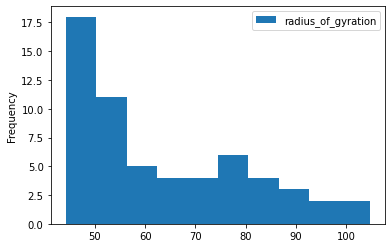To see the spatial distribution of radius of gyration, we need the geographic boundaries of the administrative regions. We can get these as GeoJSON using the FlowClient `get_geography` function, and load into a GeoPandas `GeoDataFrame` using the `GeoDataFrame.from_features` method.

``````geog = gpd.GeoDataFrame.from_features(
).set_index("pcod")
``````

The `plot` method is a quick way to see the regions described by this GeoDataFrame.

``````geog.plot();
``````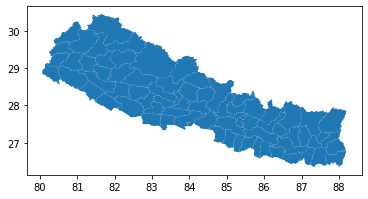Now we can join the geography data to the radius of gyration results, and create a choropleth map by plotting again using the `radius_of_gyration` column to colour the regions.
(Note: the order in which we join here is important - `rog_results.join(geog)` would produce a pandas `DataFrame`, not a geopandas `GeoDataFrame`, and the `plot` method would produce a different plot).

``````geog.join(rog_results).plot(column="radius_of_gyration", legend=True);
``````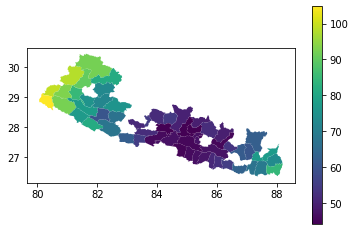There are gaps in this choropleth map. Looking at the content of the 'radius_of_gyration' column in the joined dataframe, we can see why:

``````geog.join(rog_results)[["radius_of_gyration"]].head()
``````

pcod

NPL.1.1.1_1 44.2139
NPL.1.1.2_1 49.1294
NPL.1.1.3_1 48.1
NPL.1.1.4_1 48.4194
NPL.1.1.5_1 50.7029

There are some regions for which we have no `radius_of_gyration` data, because these regions contained fewer than 15 subscribers so FlowKit redacted the results to preserve privacy. We can drop these rows using the `dropna` method. So that we can still see the shapes of the regions with no data, let's plot the `geog` data as a light grey background behind our choropleth map.

``````background = geog.plot(color="lightgrey")
geog.join(rog_results).dropna().plot(
);
``````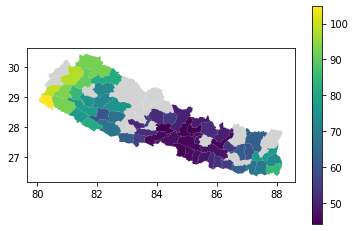### Handset type results¶

Next we get the results of our handset-type query.

``````handset_results = fc.get_result(connection=conn, query_spec=jsa_handset_query)
``````

pcod metric key value
0 NPL.1.1.1_1 value Feature 0.33871
1 NPL.1.1.1_1 value Smart 0.317618
2 NPL.1.1.1_1 value Basic 0.343672
3 NPL.1.1.2_1 value Smart 0.332919
4 NPL.1.1.2_1 value Basic 0.322981

This dataframe has four columns: `pcod` (the P-code, as above), `metric`, `key` (the handset type) and `value` (the proportion of subscribers in that region who have that handset type). The `metric` column just contains the value `"value"` in every row, which is not useful to us, so let's drop it. We'll also rename the `key` and `value` columns to `handset_type` and `proportion`, respectively.

This time we have three rows per P-code, one for each handset type, so let's set a `MultiIndex` using the `pcod` and `handset_type` columns.

``````handset_results = (
handset_results.rename(columns={"key": "handset_type", "value": "proportion"})
.drop(columns="metric")
.set_index(["pcod", "handset_type"])
)
``````

pcod, handset_type
proportion

('NPL.1.1.1_1', 'Feature') 0.33871
('NPL.1.1.1_1', 'Smart') 0.317618
('NPL.1.1.1_1', 'Basic') 0.343672
('NPL.1.1.2_1', 'Smart') 0.332919
('NPL.1.1.2_1', 'Basic') 0.322981

We can get a summary of the data using the `describe` method, but let's first group by `handset_type` to get a summary per handset type.

``````handset_results.groupby("handset_type").describe()
``````

handset_type
proportion
count

proportion
mean

proportion
std

proportion
min

proportion
25%

proportion
50%

proportion
75%

proportion
max

Basic 60 0.33332 0.0211996 0.287582 0.319938 0.333899 0.343351 0.42069
Feature 60 0.340468 0.019161 0.303725 0.330201 0.337825 0.347231 0.409677
Smart 60 0.326212 0.0239995 0.248276 0.313915 0.328385 0.340059 0.375817

To plot histograms for the three handset types, it would be convenient if the proportion data for each handset type were in separate columns. Since we set a hierarchical index on the `handset_results` dataframe, we can use the `unstack` method to unpack the innermost level of the index (`handset_type`, in this case) into separate columns.

``````handset_results.unstack().head()
``````

handset_type

pcod
proportion
Basic

proportion
Feature

proportion
Smart

NPL.1.1.1_1 0.343672 0.33871 0.317618
NPL.1.1.2_1 0.322981 0.344099 0.332919
NPL.1.1.3_1 0.337072 0.330457 0.332471
NPL.1.1.4_1 0.289941 0.337278 0.372781
NPL.1.1.5_1 0.326446 0.347107 0.326446

This produces a dataframe with a single-level index, and a `MultiIndex` as column headers. Now the `plot.hist` method plots all three histograms on the same axes. We set `alpha=0.5` to make them slightly transparent. In this dataset we can see that there is little difference between the distributions of the different handset types - the proportions of all handset types are approximately equal in all regions.

``````handset_results.unstack().plot.hist(alpha=0.5);
``````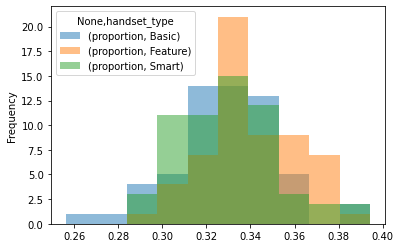As with the radius of gyration results, we can also join to the geography data and plot choropleth maps of the handset type distributions. We use the `query` method to select the subset of rows for a given handset type.

``````handset_results_with_geo = geog.join(handset_results).dropna()

for htype in ["Basic", "Feature", "Smart"]:
ax = geog.plot(color="lightgrey")
ax.set_title(f"Proportion ({htype})")
handset_results_with_geo.query(f"handset_type == '{htype}'").plot(
ax=ax, column="proportion", legend=True
);
``````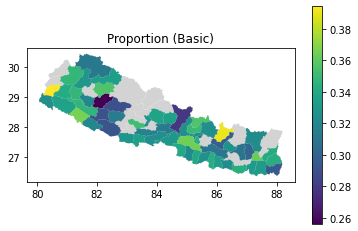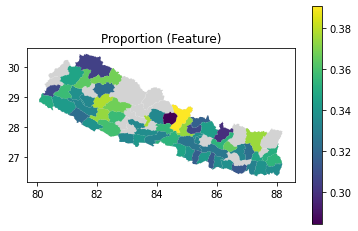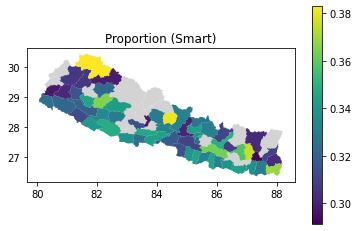### Comparing radius of gyration and handset types¶

#### Join the dataframes¶

Now that we have the results from both of our joined spatial aggregate queries, we can join them and compare the metrics.

``````combined = rog_results.join(handset_results.unstack())
``````

pcod

('proportion', 'Basic')

('proportion', 'Feature')

('proportion', 'Smart')

NPL.1.3.3_1 49.1169 0.313158 0.342105 0.344737
NPL.4.1.1_1 75.0083 0.344605 0.336554 0.318841
NPL.1.1.3_1 48.1 0.337072 0.330457 0.332471
NPL.3.1.4_1 107.041 0.341525 0.344675 0.3138
NPL.1.2.1_1 54.3766 0.34273 0.345697 0.311573

#### Correlation¶

The `corr` method provides a quick way to calculate the correlation between all pairs of columns. We're interested in the correlation between radius of gyration and each of the proportion columns. From these results, there appears to be no significant correlation between handset type and radius of gyration.

``````combined.corr()
``````
radius_of_gyration ('proportion', 'Basic') ('proportion', 'Feature') ('proportion', 'Smart')
('proportion', 'Basic') -0.17396 1 -0.296146 -0.646895
('proportion', 'Feature') -0.0648309 -0.296146 1 -0.536797
('proportion', 'Smart') 0.205425 -0.646895 -0.536797 1

#### Scatter plot¶

To see whether there is any relationship that's not apparent from the correlation, let's make a scatter plot of radius of gyration against proportion of subscribers who use a smartphone.

``````combined.plot.scatter(x="radius_of_gyration", y=("proportion", "Smart"));
``````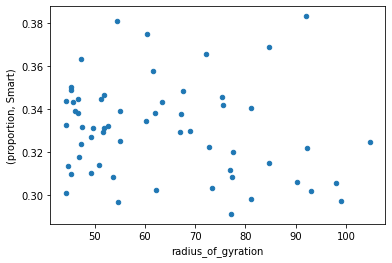We can use `matplotlib` to plot radius of gyration against proportion for each of the handset types on the same plot.

``````fig, ax = plt.subplots()

for htype in ["Basic", "Feature", "Smart"]:
ax.scatter(